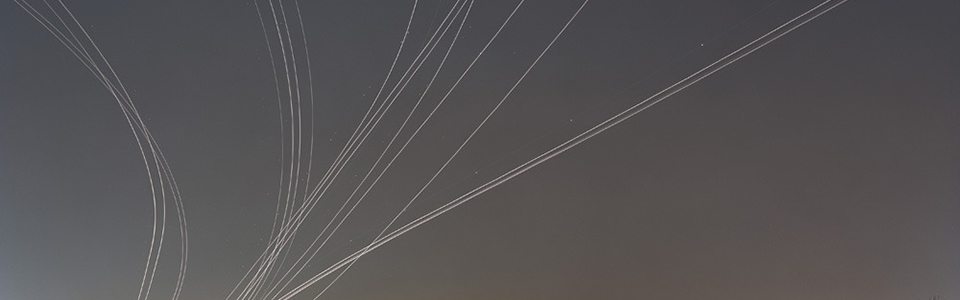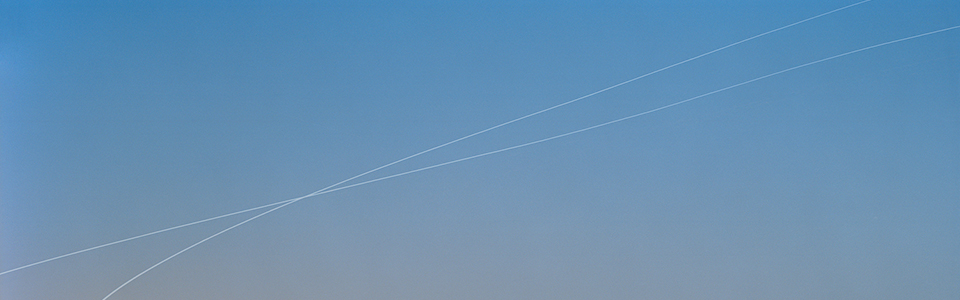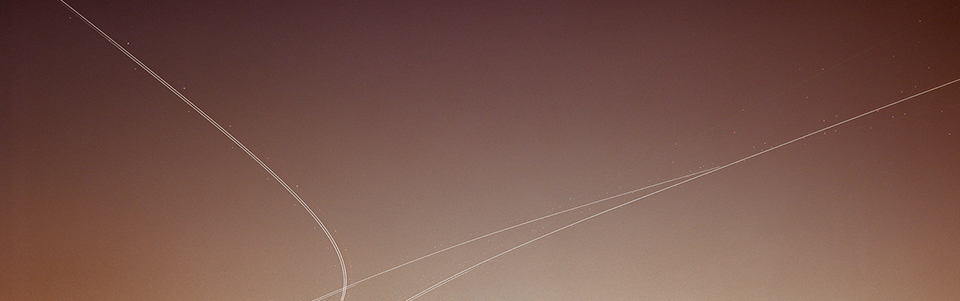I conduct research activity in optimal control of hybrid dynamic systems with applications to robotics, aeronautics, and astronautics. Hybrid dynamical systems are dynamical systems that have both continuous and discrete dynamic behavior. Therefore, the state of a hybrid system is characterized by continuous and discrete state variables which are called modes. In this problem the goal is to find the continuous control inputs, the sequence of modes, and the corresponding trajectory that steer the system between two states satisfying a set of constraints on the state and/or control variables while optimizing an objective functional. Typically, optimal control problems for hybrid dynamical systems are highly non-linear and it is very difficult to find analytical solutions even for the simplest cases. The common practice is to use numerical methods to get approximate solutions.